CBSE Class 10 Sample Paper for 2020 Boards - Maths Standard

Class 10
Solutions of Sample Papers for Class 10 Boards

## The perimeters of two similar triangles ∆ABC and ∆PQR are 35 cm & 45 cm respectively, then the ratio of the areas of the two triangles is______________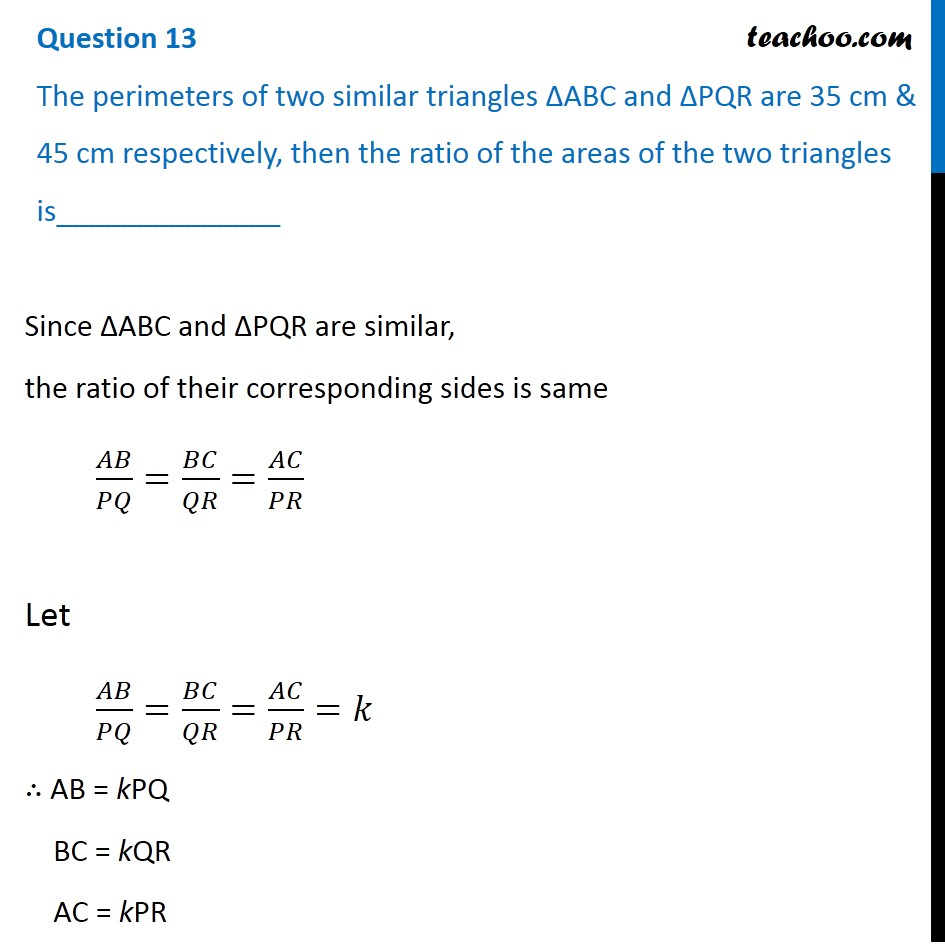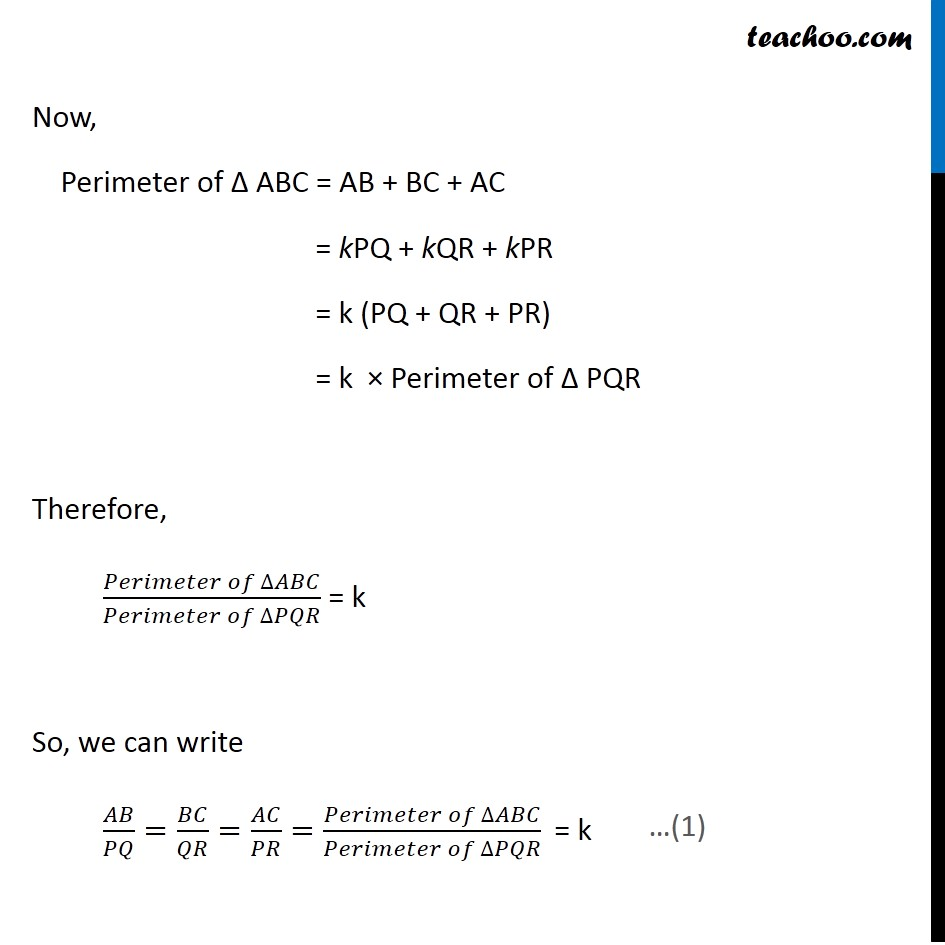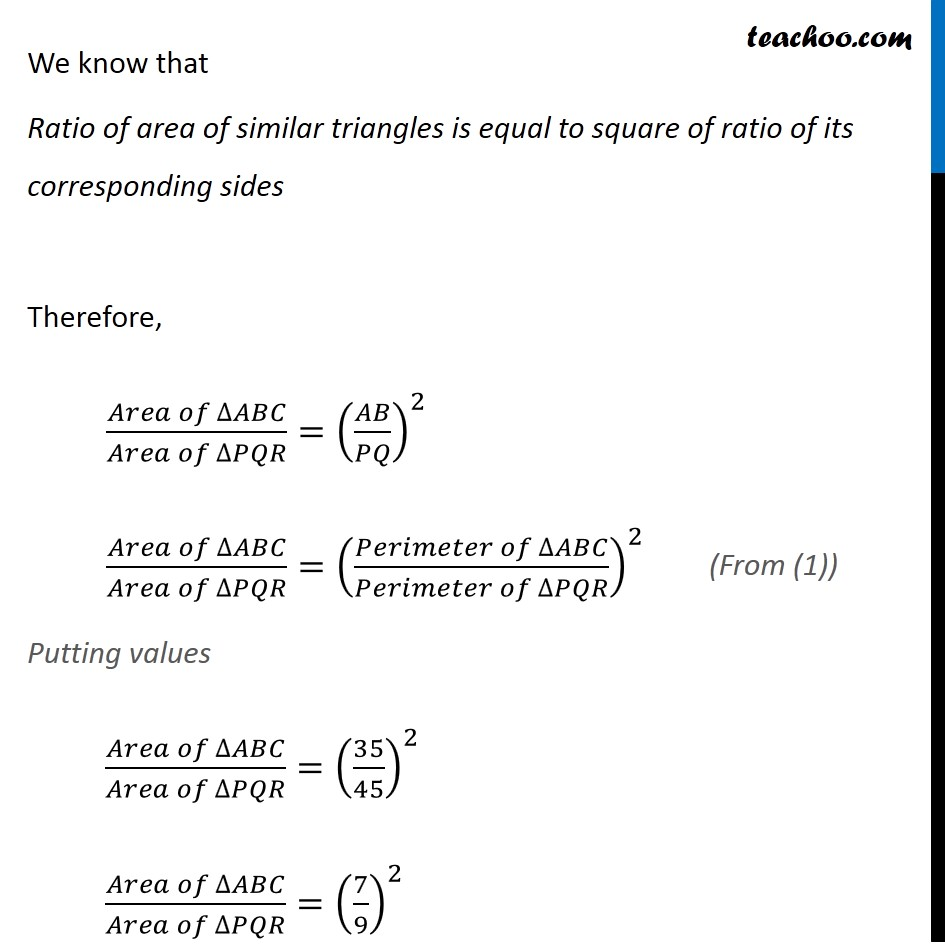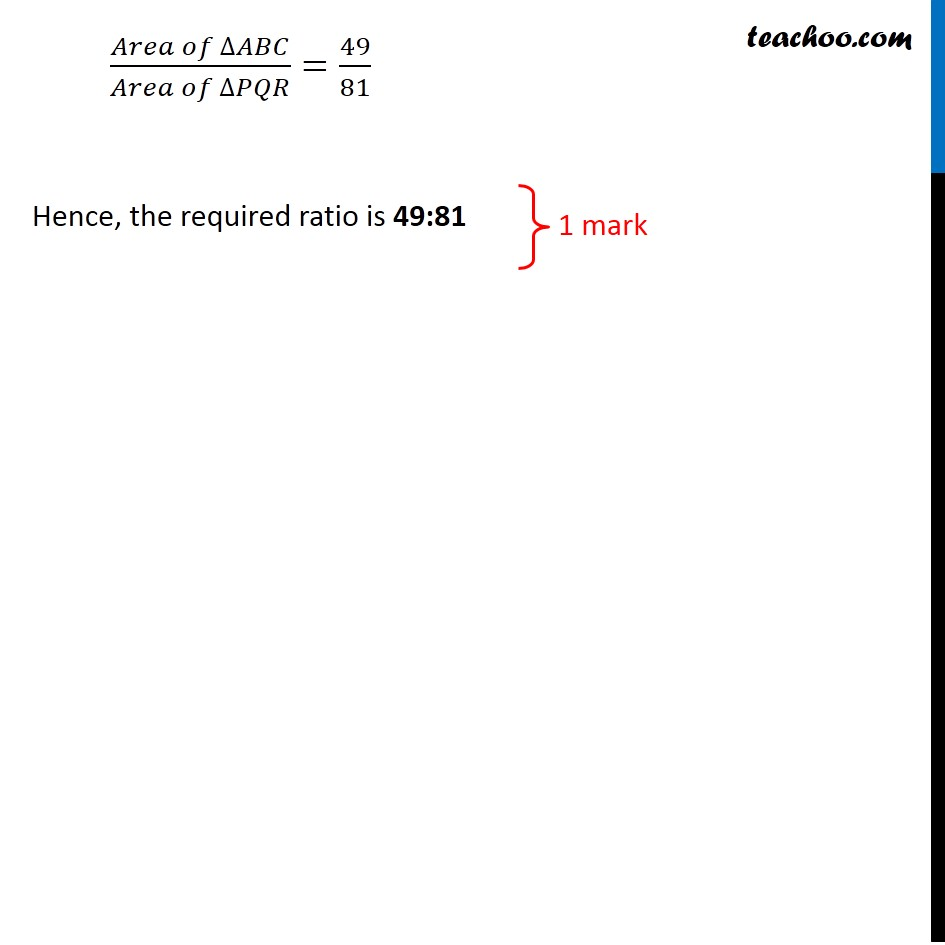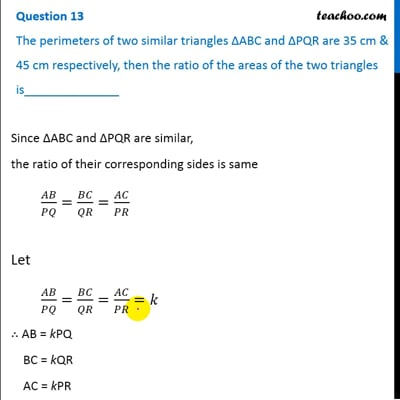This video is only available for Teachoo black users

### Transcript

Question 13 The perimeters of two similar triangles ∆ABC and ∆PQR are 35 cm & 45 cm respectively, then the ratio of the areas of the two triangles is______________ Since ΔABC and ΔPQR are similar, the ratio of their corresponding sides is same 𝐴𝐵/𝑃𝑄=𝐵𝐶/𝑄𝑅=𝐴𝐶/𝑃𝑅 Let 𝐴𝐵/𝑃𝑄=𝐵𝐶/𝑄𝑅=𝐴𝐶/𝑃𝑅=𝑘 ∴ AB = kPQ BC = kQR AC = kPR Now, Perimeter of Δ ABC = AB + BC + AC = kPQ + kQR + kPR = k (PQ + QR + PR) = k × Perimeter of Δ PQR Therefore, (𝑃𝑒𝑟𝑖𝑚𝑒𝑡𝑒𝑟 𝑜𝑓" " ∆𝐴𝐵𝐶)/(𝑃𝑒𝑟𝑖𝑚𝑒𝑡𝑒𝑟 𝑜𝑓" " ∆𝑃𝑄𝑅) = k So, we can write 𝐴𝐵/𝑃𝑄=𝐵𝐶/𝑄𝑅=𝐴𝐶/𝑃𝑅=(𝑃𝑒𝑟𝑖𝑚𝑒𝑡𝑒𝑟 𝑜𝑓" " ∆𝐴𝐵𝐶)/(𝑃𝑒𝑟𝑖𝑚𝑒𝑡𝑒𝑟 𝑜𝑓" " ∆𝑃𝑄𝑅) " = k " We know that Ratio of area of similar triangles is equal to square of ratio of its corresponding sides Therefore, (𝐴𝑟𝑒𝑎 𝑜𝑓" " ∆𝐴𝐵𝐶)/(𝐴𝑟𝑒𝑎 𝑜𝑓" " ∆𝑃𝑄𝑅)=(𝐴𝐵/𝑃𝑄)^2 (𝐴𝑟𝑒𝑎 𝑜𝑓" " ∆𝐴𝐵𝐶)/(𝐴𝑟𝑒𝑎 𝑜𝑓" " ∆𝑃𝑄𝑅)=((𝑃𝑒𝑟𝑖𝑚𝑒𝑡𝑒𝑟 𝑜𝑓" " ∆𝐴𝐵𝐶)/(𝑃𝑒𝑟𝑖𝑚𝑒𝑡𝑒𝑟 𝑜𝑓" " ∆𝑃𝑄𝑅))^2 Putting values (𝐴𝑟𝑒𝑎 𝑜𝑓" " ∆𝐴𝐵𝐶)/(𝐴𝑟𝑒𝑎 𝑜𝑓" " ∆𝑃𝑄𝑅)=(35/45)^2 (𝐴𝑟𝑒𝑎 𝑜𝑓" " ∆𝐴𝐵𝐶)/(𝐴𝑟𝑒𝑎 𝑜𝑓" " ∆𝑃𝑄𝑅)=(7/9)^2 (𝐴𝑟𝑒𝑎 𝑜𝑓" " ∆𝐴𝐵𝐶)/(𝐴𝑟𝑒𝑎 𝑜𝑓" " ∆𝑃𝑄𝑅)=49/81 Hence, the required ratio is 49:81